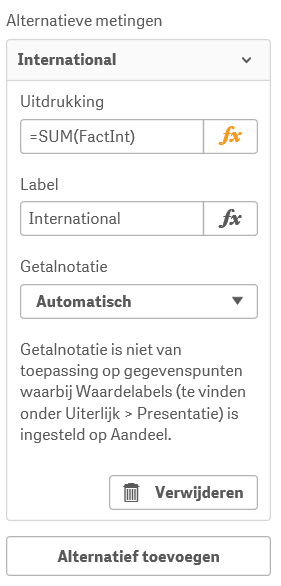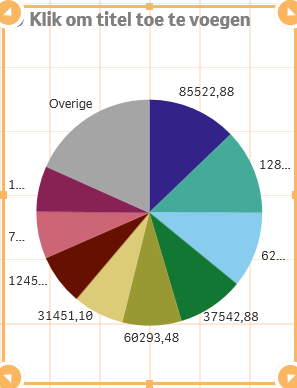# App Development

Announcements
cancel
Showing results for
Did you mean:Contributor III

## Convert QLikView pie-chart with two expressions to QLikSense pie chart

First I made a pie-chart in QLikView that has two expressions but no dimension. The two expression in the chart are: =SUM(FactNat) and =SUM(FactInt).

I tried to create the same chart in QLikSense but my problem is that QLikSense obliged me to create a dimension that the current pie chart doesn't contain.My pie-chart in QLikViewWhen I added the two expressions to the pie-chart, I don't get a visualization. What do I need to put as dimension to the pie-chart?

I tried to add FactInt as dimension that results to:And FactNat results to:How do I get the pie-chart shown in QLikView?

1 Solution

Accepted SolutionsEmployee

Try this:

As a calculated dimension:

=ValueList('FactNat','FactInt')

if(ValueList('FactNat','FactInt')='FactNat',sum(FactNat),

if(ValueList('FactNat','FactInt')='FactInt',sum(FactInt)))

You will need to do some conditional colouring and labelling

2 RepliesEmployee

Try this:

As a calculated dimension:

=ValueList('FactNat','FactInt')

if(ValueList('FactNat','FactInt')='FactNat',sum(FactNat),

if(ValueList('FactNat','FactInt')='FactInt',sum(FactInt)))

You will need to do some conditional colouring and labellingContributor III
Author

This is exactly what I needed, thanks.